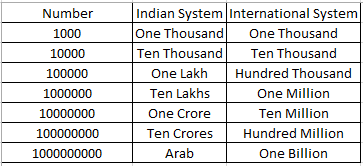Courses
Courses for Kids
Free study material
Free LIVE classes
MoreLIVE
Join Vedantu’s FREE Mastercalss

# 100 million is equal to$\begin{gathered} {\text{A}}{\text{. 1 crore}} \\ {\text{B}}{\text{. 10 lakh}} \\ {\text{C}}{\text{. 10 crore}} \\ {\text{D}}{\text{. 100 crore}} \\ \end{gathered}$Verified
262.8k+ views
Hint- In this question we have to convert the given number from international number system to Indian number system. Draw a table for the conversion of the same and analyze it.
In this question we have to convert this given number from the international system to Indian number system.
First, we should draw a table of conversion from international number system to Indian number system.As, we can see here that One thousand in Indian system is equal to one thousand in the international system.
Similarly, ten thousand is equal to ten thousand in international system, one lakh is equal to hundred thousand in international system, ten lakhs are equal to one million in international system, one crore is equal to ten million in international system and ten crores is equal to hundred million in international system.
So, 100 million is equal to 10 crores, which is option C.

Note- Whenever we face such types of problems the key point to remember is that we need to have a good grasp over the conversion of Indian number system to international number system, you can also make a table, like drawn above which can help you in simplification and getting on the right track to reach the answer.
Last updated date: 23rd Sep 2023
Total views: 262.8k
Views today: 2.62k